# 4. The following function is given: OUT = A + B. (C + D + E) Design a singk-stage CMOS circuit wi...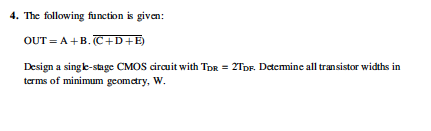I'm new to the subject. Please explain the steps where possible.

4. The following function is given: OUT = A + B. (C + D + E) Design a singk-stage CMOS circuit with Tor 2TDF Determine all transistor widths in terms of minimum geometry, W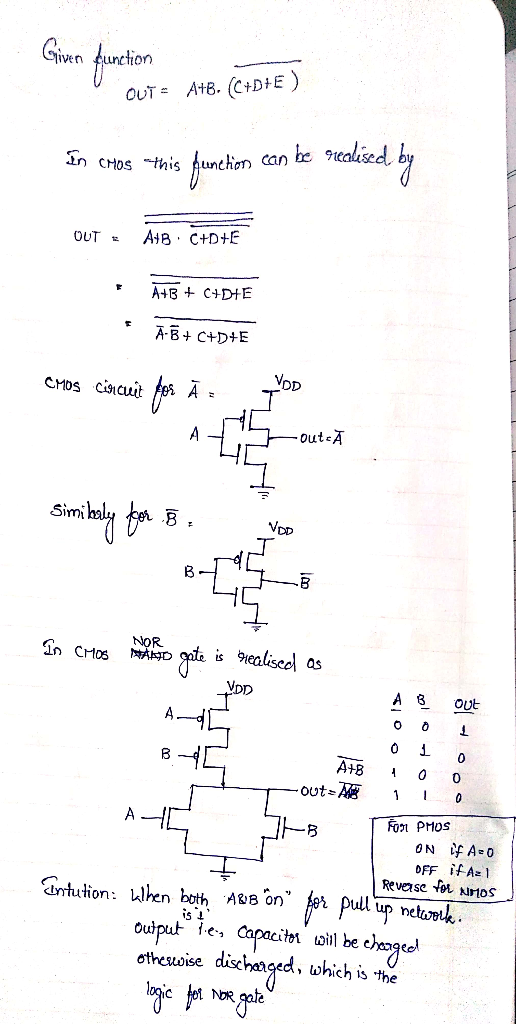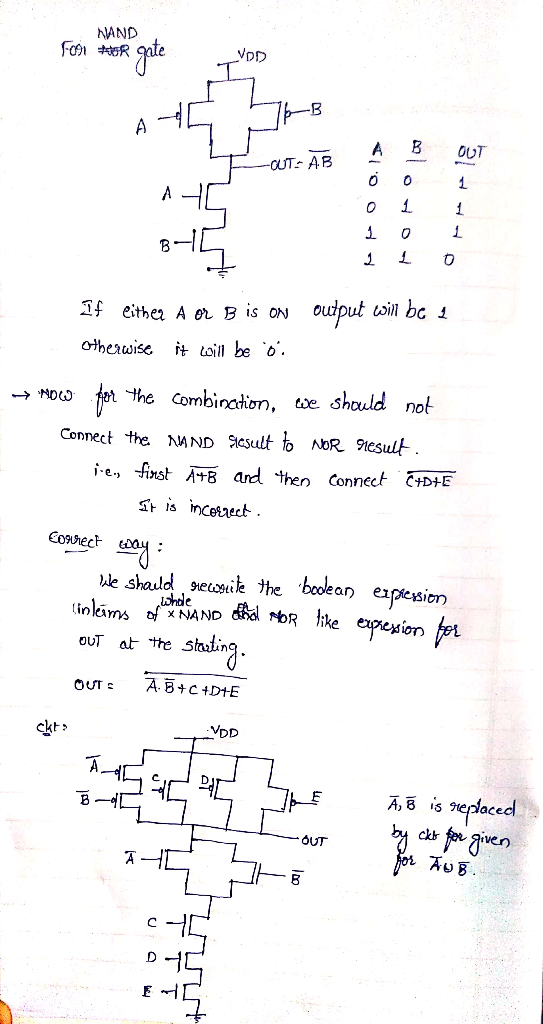##### Add Answer of: 4. The following function is given: OUT = A + B. (C + D + E) Design a singk-stage CMOS circuit wi...
Similar Homework Help Questions
• ### 3. Design the CMOS gate that implements the following function OUT- AB (a) Draw the circuit schematic without any output inverter. (b) Size all the transistors in this circuit such that TR 3Tr. The m...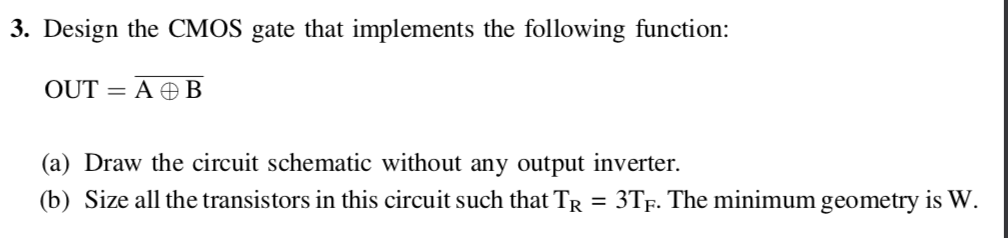3. Design the CMOS gate that implements the following function OUT- AB (a) Draw the circuit schematic without any output inverter. (b) Size all the transistors in this circuit such that TR 3Tr. The minimum geometry is W. 3. Design the CMOS gate that implements the following function OUT- AB (a) Draw the circuit schematic without any output inverter. (b) Size all the transistors in this circuit such that TR 3Tr. The minimum geometry is W.

• ### Problem 4 Design the static complementary CMOS implementation of a 2-bit comparator circuit, wher...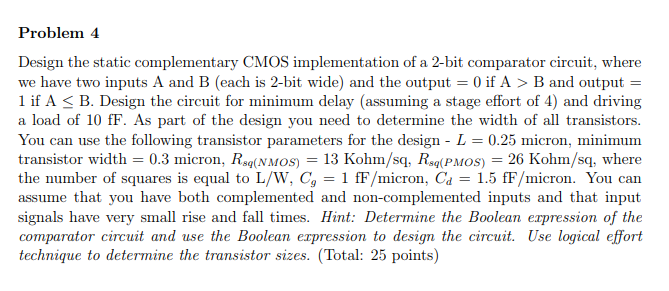Problem 4 Design the static complementary CMOS implementation of a 2-bit comparator circuit, where we have two inputs A and B (each is 2-bit wide) and the output 0 if A > B and output 1 if A B. Design the circuit for minimum delay (assuming a stage effort of 4) and driving a load of 10 fF. As part of the design you need to determine the width of all transistors You can use the following transistor parameters for...

• ### The layout of a CMOS complex logic circuit is given in the Figure 1. 4. Draw the corresponding circuit diagram; and (10 Marks) a. b. Colculate the W/Doivalent of all the nMOS and PMOS transistors...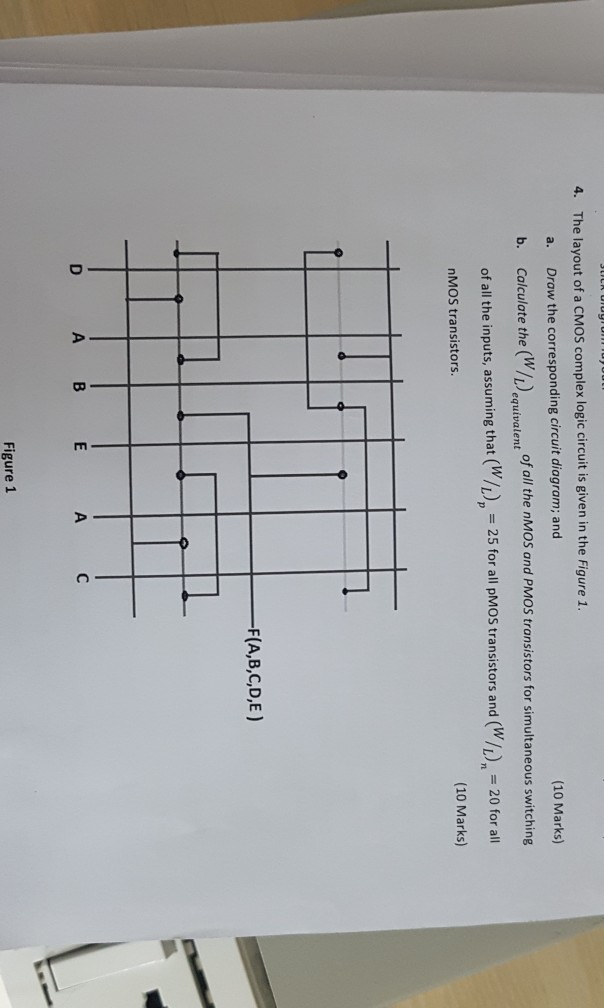The layout of a CMOS complex logic circuit is given in the Figure 1. 4. Draw the corresponding circuit diagram; and (10 Marks) a. b. Colculate the W/Doivalent of all the nMOS and PMOS transistors for simultaneous switching of all the inputs, assuming that (W/, 25 for all MOS transistors and (W/, 20 for al nMOS transistors. (10 Marks) FIA, B,C,D,E ) A B Figure 1 The layout of a CMOS complex logic circuit is given in the Figure 1....

• ### The layout of a CMOS complex logic circuit is given in the Figure 1. 1. Draw the corresponding circuit diagram; and a....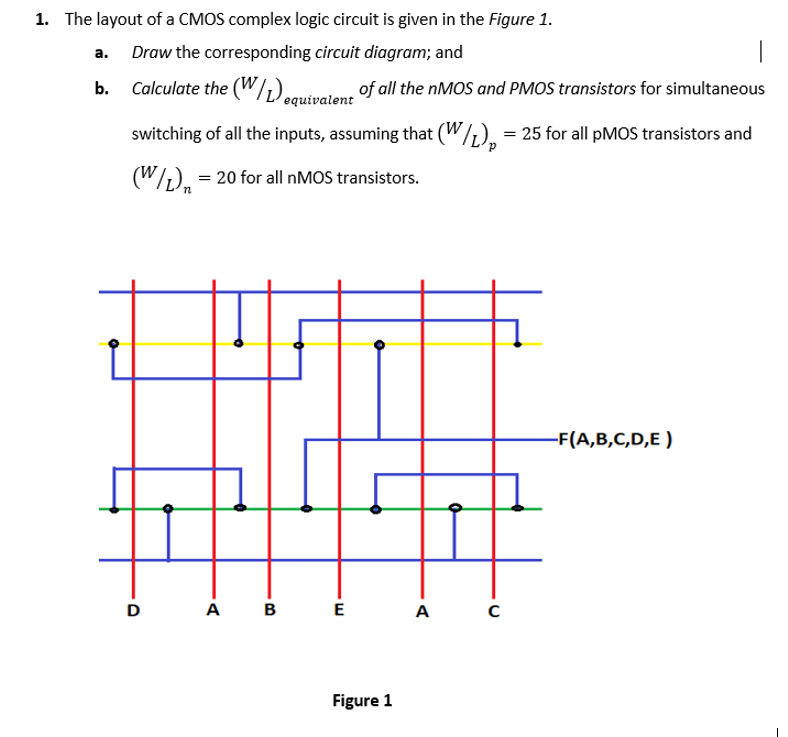The layout of a CMOS complex logic circuit is given in the Figure 1. 1. Draw the corresponding circuit diagram; and a. b. Calculate the (W/equivaientfall the nMOS and PMOS transistors for simultaneous equivalent switching of all the inputs, assuming that (W/L), = 25 for all pMOS transistors and W-20 for all nMOS transistors F(A,B,C,D,E ) A B Figure 1 The layout of a CMOS complex logic circuit is given in the Figure 1. 1. Draw the corresponding circuit diagram;...

• ### 1.5.5 In Class Exercise: Work out the following examples from the text. Design CMOS logic functio...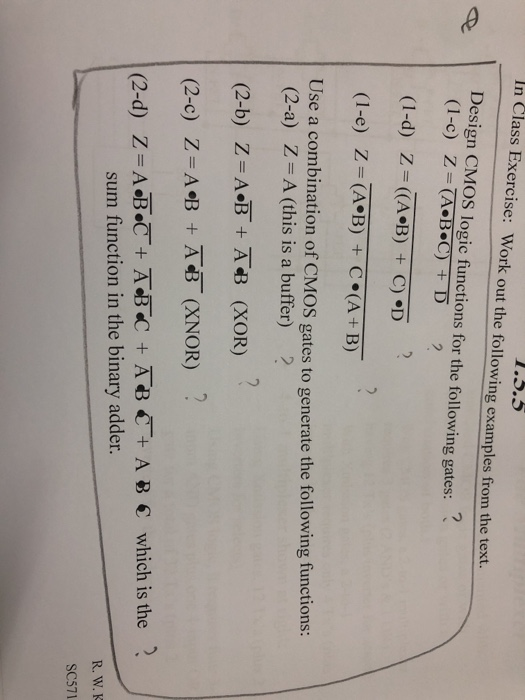1.5.5 In Class Exercise: Work out the following examples from the text. Design CMOS logic functions for the following gates: (1-e) Z = (A·B) C.(A+ B) + Use a combination of CMOS gates to generate the following functions (2-a) Z A (this is a buffer) (2-c) Z- A B A (XNOR)? (2-d) Z-AbeT+AnB C +ABC + AB € which is the ? sum function in the binary adder. SC571 1.5.5 In Class Exercise: Work out the following examples from the...

• ### a. Design and implement a combinational circuit with four inputs w,x, y and z and four outputs A, B,C and D using CMOS...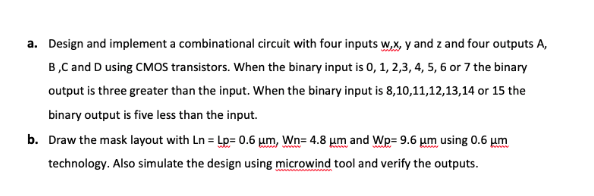a. Design and implement a combinational circuit with four inputs w,x, y and z and four outputs A, B,C and D using CMOS transistors. When the binary input is 0, 1, 2,3, 4, 5, 6 or 7 the binary output is three greater than the input. When the binary input is 8,10,11,12,13,14 or 15 the binary output is five less than the input. b. Draw the mask layout with Ln Lp 0.6 um, Wn- 4.8 um and Wp- 9.6 um...

• ### a. Design and implement a combinational circuit with four inputs w,x, y and z and four outputs A, B,C and D using CMOS...a. Design and implement a combinational circuit with four inputs w,x, y and z and four outputs A, B,C and D using CMOS transistors. When the binary input is 0, 1, 2,3, 4, 5, 6 or 7 the binary output is three greater than the input. When the binary input is 8,10,11,12,13,14 or 15 the binary output is five less than the input. b. Draw the mask layout with Ln Lp 0.6 um, Wn- 4.8 um and Wp- 9.6 um...

• ### Please answer every part 1) Six Transistor CMOS Logic Circuit, Z-output; A, B, C are the inputs. 15 pts The three P-devices are connected as follows: Q2S-5V; Q2D-Q4S Q6S; Q4D-Q6D-Z. The three N-devic...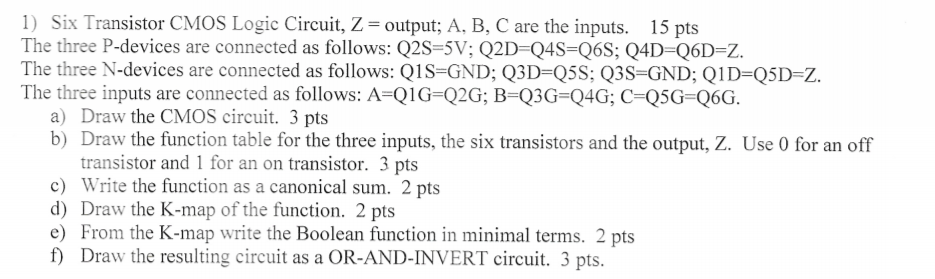Please answer every part 1) Six Transistor CMOS Logic Circuit, Z-output; A, B, C are the inputs. 15 pts The three P-devices are connected as follows: Q2S-5V; Q2D-Q4S Q6S; Q4D-Q6D-Z. The three N-devices are connected as follows: QiS-GND Q3D-Q5S Q3S GNDQID-Q5D-Z The three inputs are connected as follows: A-QIG-Q2G; B-Q3G-Q4G; C Q5G Q6G. a) Draw the CMOS circuit. 3 pts b) Draw the function table for the three inputs, the six transistors and the output, Ζ. Use 0 for an...

• ### The layout of a CMOS complex logic circuit is given in the Figure 1 4. Draw the corresponding circuit diagram; and (10 Marks) a. b. Calculate the (W) of all the nMOS and PMOS transistors for simul...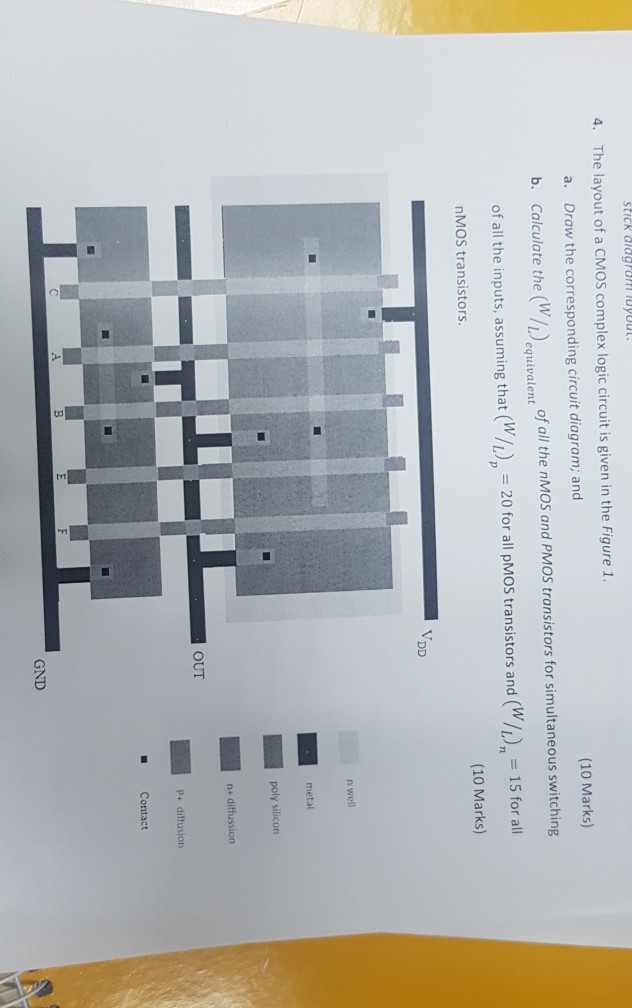The layout of a CMOS complex logic circuit is given in the Figure 1 4. Draw the corresponding circuit diagram; and (10 Marks) a. b. Calculate the (W) of all the nMOS and PMOS transistors for simultaneous switching (W/), 15 for all of all the inputs, assuming that (Wh),-20 for all pMOS transistors and (w/L), = 15 for all (WL 20 for all pMOS transistors and (10 Marks) nMOS transistors VDD n well metal poly silicon n+ diffussion OUT Contact...

• ### Design and implement a combinational circuit with four inputs w,x, y and z and four outputs A, B ,C and D using CMOS transistors. When the binary input is 0, 1, 2,3,4,5,6 or 7 the binary output is fi...

Design and implement a combinational circuit with four inputs w,x, y and z and four outputs A, B ,C and D using CMOS transistors. When the binary input is 0, 1, 2,3,4,5,6 or 7 the binary output is five greater than the input. When the binary input is 8,,10,11,12,13,14 or 15 the binary output is seven less than the input. for question (a) find the troth table for the inputs (ABCD) then implement using K-map to find the equations to...

Need Online Homework Help?